Question

Solve differential equation with initial condition. Final answer in form y=f(x). Please show all steps, thank you!!!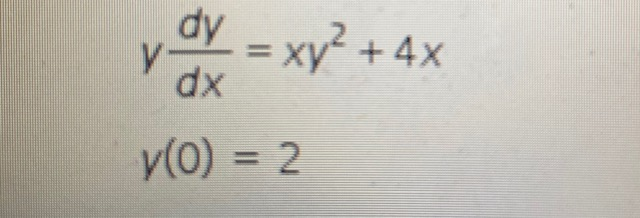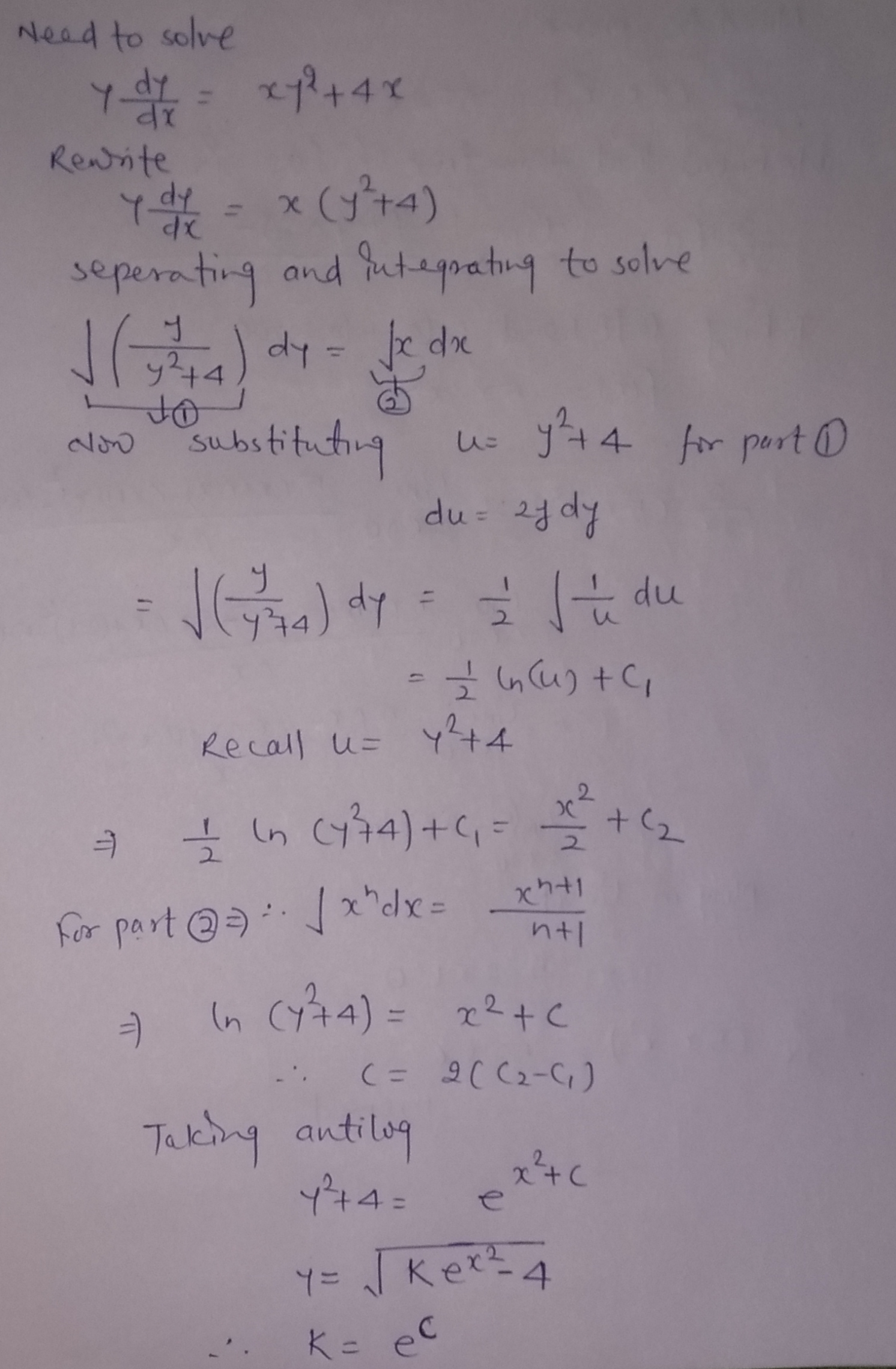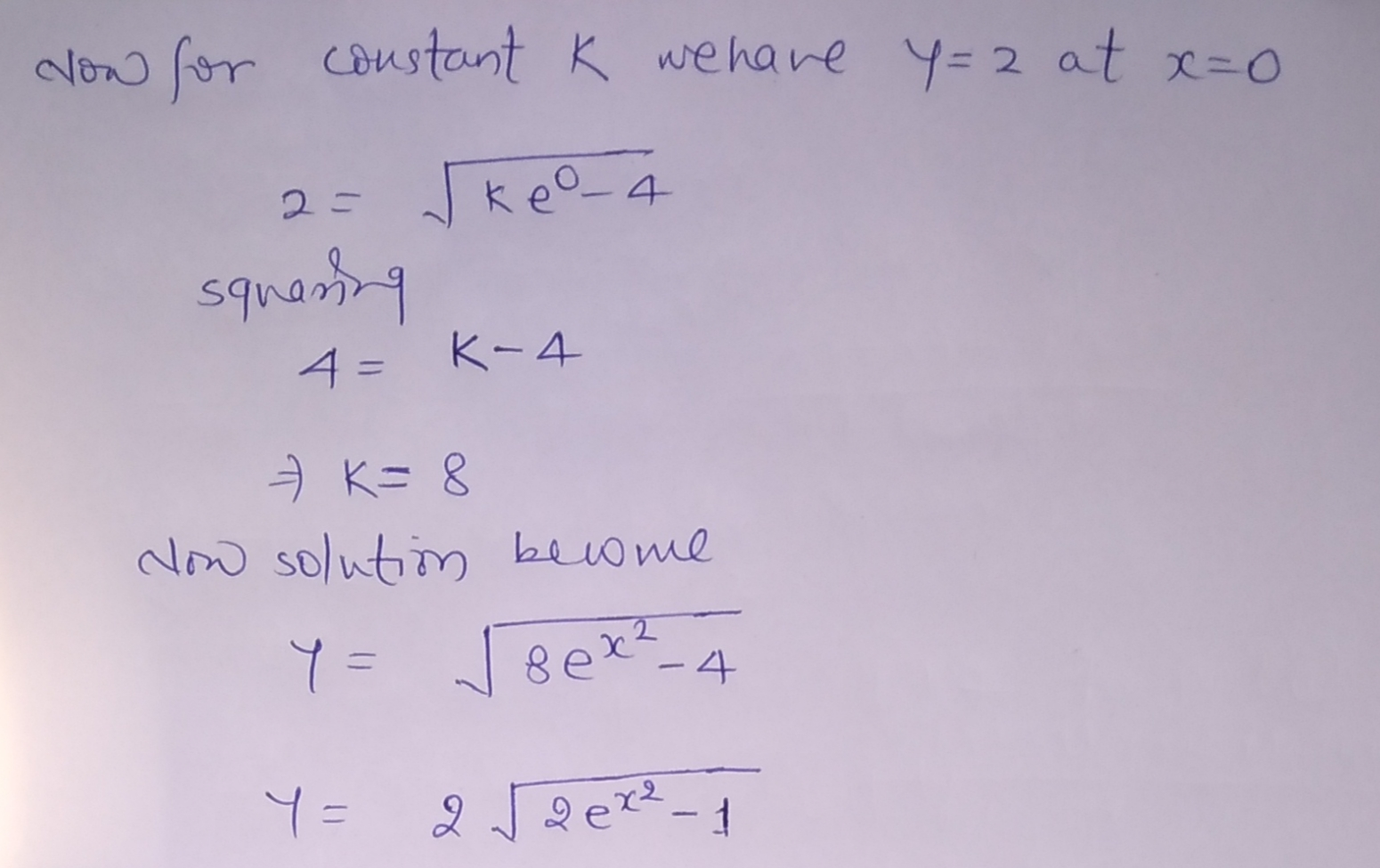#### Earn Coins

Coins can be redeemed for fabulous gifts.

Similar Homework Help Questions
• ### Please show all the steps of these questions. Solve the differential equation y' + y cos...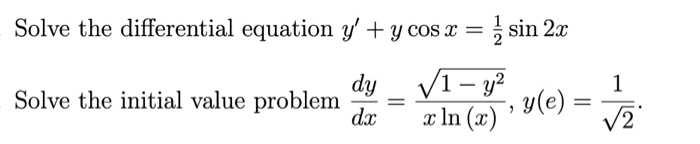Please show all the steps of these questions. Solve the differential equation y' + y cos x = { sin 2x dy V1 - y2 Solve the initial value problem y(e) = dx x In (x) 1 = V2

• ### 5. Find the solution of the differential equation that satisfies the given initial condition dy cos' xsin dx ysin y Yo) - 1. Leave the answer in the implici form. ,y(o)- 1. Leave the answer i...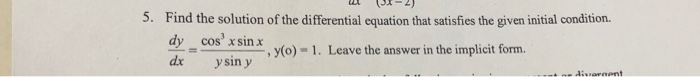5. Find the solution of the differential equation that satisfies the given initial condition dy cos' xsin dx ysin y Yo) - 1. Leave the answer in the implici form. ,y(o)- 1. Leave the answer in the implicit form. 5. Find the solution of the differential equation that satisfies the given initial condition dy cos' xsin dx ysin y Yo) - 1. Leave the answer in the implici form. ,y(o)- 1. Leave the answer in the implicit form.

• ### With 5. Consider the differential equation y, f(x,y) with initial condition y(zo) = yo. Show that...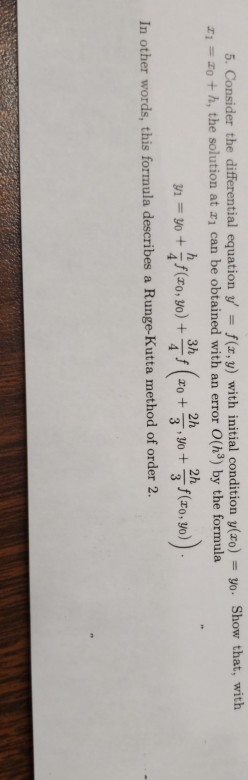1 with 5. Consider the differential equation y, f(x,y) with initial condition y(zo) = yo. Show that, zi = zo +h, the solution at x1 can be obtained with an er ror O(h3) by the formula In other words, this formula describes a Runge-Kutta method of order 2. with 5. Consider the differential equation y, f(x,y) with initial condition y(zo) = yo. Show that, zi = zo +h, the solution at x1 can be obtained with an er ror O(h3)...

• ### Find a function y=f(x) satisfying the given differential equation and the prescribed initial condition. 1 dy...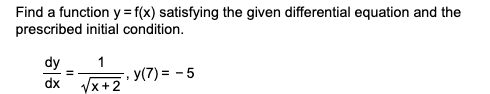Find a function y=f(x) satisfying the given differential equation and the prescribed initial condition. 1 dy dx y(7) = -5 1x + 2

• ### Please show steps thank you Find the derivative of y with respect to x if y...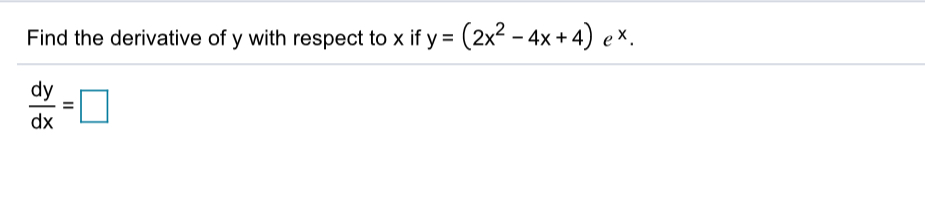Please show steps thank you Find the derivative of y with respect to x if y = (2x2 - 4x +4) ex dy dx =

• ### Solve the differential equation given the initial condition provided. Do not solve explicity for y. =...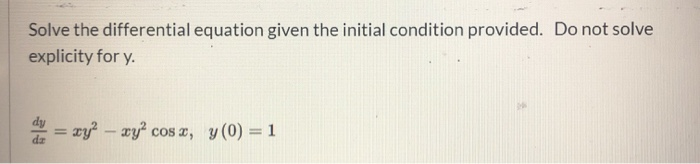Solve the differential equation given the initial condition provided. Do not solve explicity for y. = xy? – xy” cos x, y(0) = 1

• ### Please explain clearly and show all steps. Thank you. Solve the following first order equation 2x...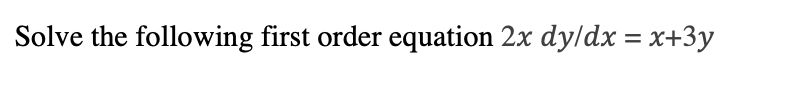Please explain clearly and show all steps. Thank you. Solve the following first order equation 2x dy/dx = x+3y

• ### Solve the given linear differential equation subject to the indicated initial condition. dy 1 -y= xe*...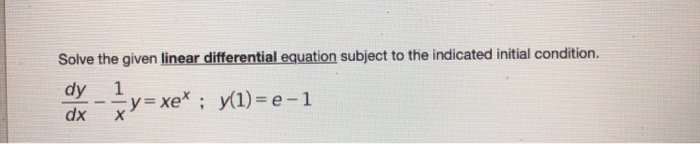Solve the given linear differential equation subject to the indicated initial condition. dy 1 -y= xe* ; y1)= e-1 dx х y= xe" ;

• ### Show all steps please. b. ASSUME the Differential Equation: dx dt :-(W2)x. 1. SOLVE. That is,...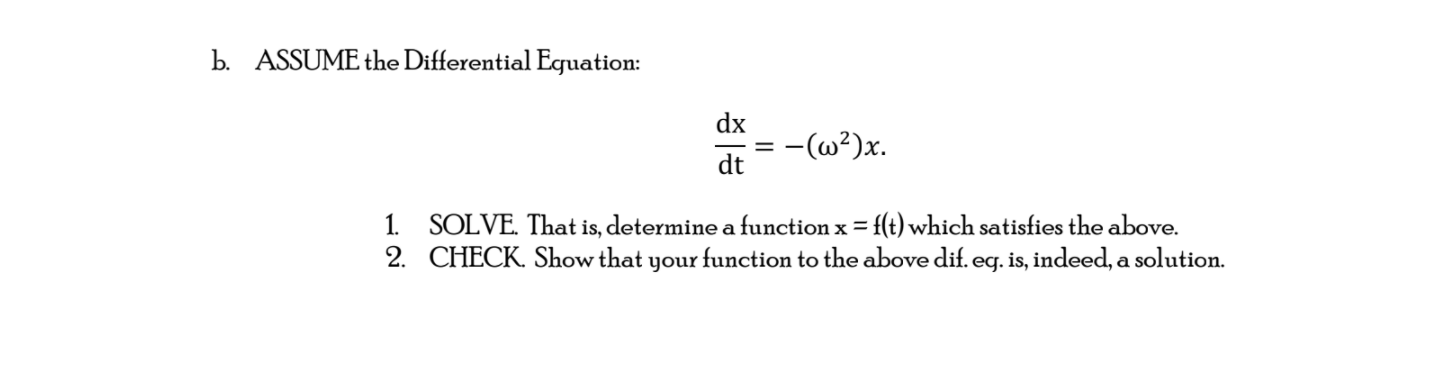Show all steps please. b. ASSUME the Differential Equation: dx dt :-(W2)x. 1. SOLVE. That is, determine a function x = f(t) which satisfies the above. 2. CHECK. Show that your function to the above dif. eg. is, indeed, a solution.

• ### Please help (1 point) In this problem we consider an equation in differential form M dx...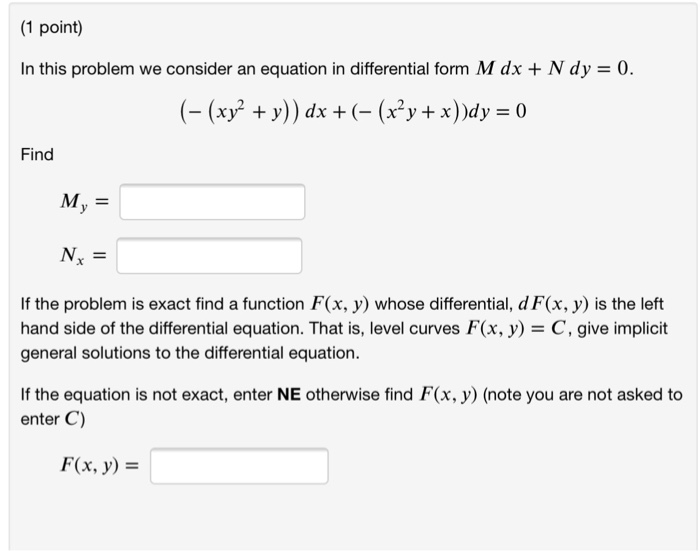Please help (1 point) In this problem we consider an equation in differential form M dx + N dy-0 (- (xy' +y)) dx + (- (x2y + x))dy 0 Find If the problem is exact find a function F(x, y) whose differential, d F(x, y) is the left hand side of the differential equation. That is, level curves F(x, y C, give implicit general solutions to the differential equation. If the equation is not exact, enter NE otherwise find F(x,...